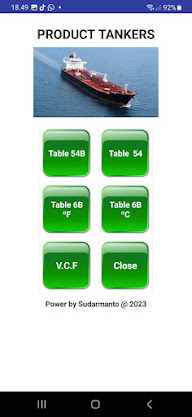## Selasa, 28 Mei 2013

### Partially Filled Spherical tank Calculator

Computing the volume of liquid in a partially-filled spherical tank is rather
complex because the volume formulas are more involved than the simple
sphere volume formula.

For ease of calculating, we chose
a) liquid level is height ( h ) and
b) tank radius = r  or ( diameter /2) as the data items required for input.

If you only know cap height and diameter, just remember this simple relationship:
Liquid Level = Diameter - Cap Height.

The total volume of a partially-filled spherical tank equals total sphere volume
minus spherical cap volume

Formula for this calculation :
Okay,... lets try calculate, for example  :
liquid level / height ( h ) = 180 cm
Tank radius ( r )  = 120 cm
How much Liquid volume ?

Volume = ( Pi * h^2 * r  ) - ( Pi * h^3 / 3 )
V  = ( 3.14 x 180 ^2 x 120 ) - ( 3.14 x 180 ^3 / 3 )
V = ( 3.14 x 32400 x 120 ) - ( 3.14 x 5832000 / 3 )
= 12214512.237  -  6107256.119
= 6107256.118  : 1000
= 6107.3  litre

to quickly calculate, please see screensooht below :

Use this tool to quickly calculate the volume of liquid in a spherical storage tank
based on the radius of the tank and the height of the liquid in the tank

Luckily, this calculator is very easy to use and will compute those
volumes for you.
The only confusion that could arise is that this is for a spherical tank.
(Basically a perfect sphere).

Okay, ...... see you on the next post

### Product Tankers 9W

Product Tankers 9W PRODUCT TANKERS 9W adalah aplikasi sederhana hanya untuk android, Aplikasi ini mengacu pada aplikasi sebelumnya, dengan...